Function Repository Resource:

# IteratedAffinePlot

Plot polygons after iteratively applying a translation, scaling and rotation

 ResourceFunction["IteratedAffinePlot"][rules, init] plots the polygon described by the points init, and the transformed shape after applying the transformation given by rules. ResourceFunction["IteratedAffinePlot"][rules, init,iter] plots the polygon described by the points init, and the transformed shape after applying the transformation given by rules, for the number of iterations specified by iter.

## Details and Options

init is a list of coordinates describing the initial polygon.
rules is a list of the form {{{x1,y1},s1,θ1},}, where {xi,yi} specifies the coordinates of the centroid of the transformed polygons, si specifies the scaling factors and θi specifies the rotation angles.
iter plots the transformed shapes for the number of iterations as given by iter. It is an optional argument, with the default value being 1. It can take any of the following forms:
 n all iterations through n {{n}} plot only the nth iteration {n1,n2} plots iterations from n1 through n2 {n1,n2,dn} plots iterations from n1 through n2 in steps of dn
ResourceFunction["IteratedAffinePlot"] accepts all options of Graphics, with the additional option "ShowMarker".
With the default "ShowMarker"True, markers showing the orientation of the transformed polygons are shown.

## Examples

### Basic Examples (1)

Show a single iteration of a given set of transformation rules, along with the initial polygon:

 In:=Out=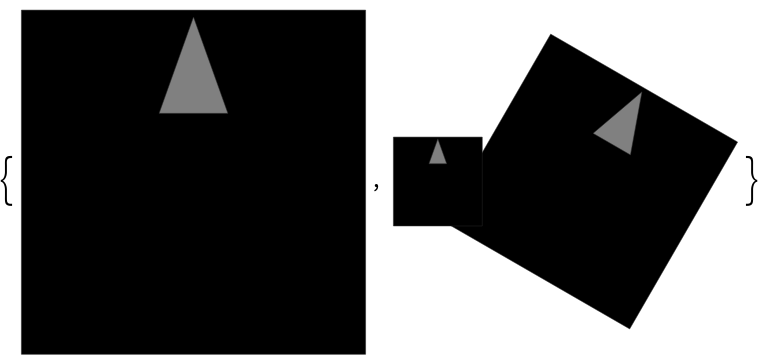### Scope (2)

Show 5 iterations of a set of transformation rules:

 In:=Out=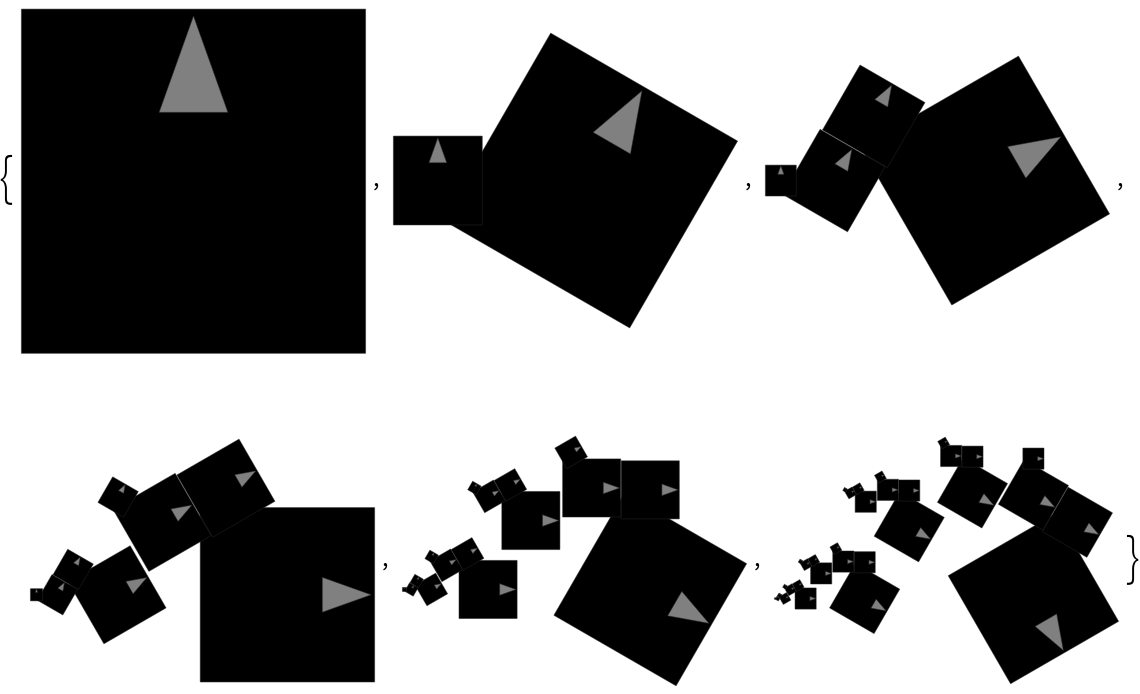Show the fifth through tenth iterations:

 In:=Out=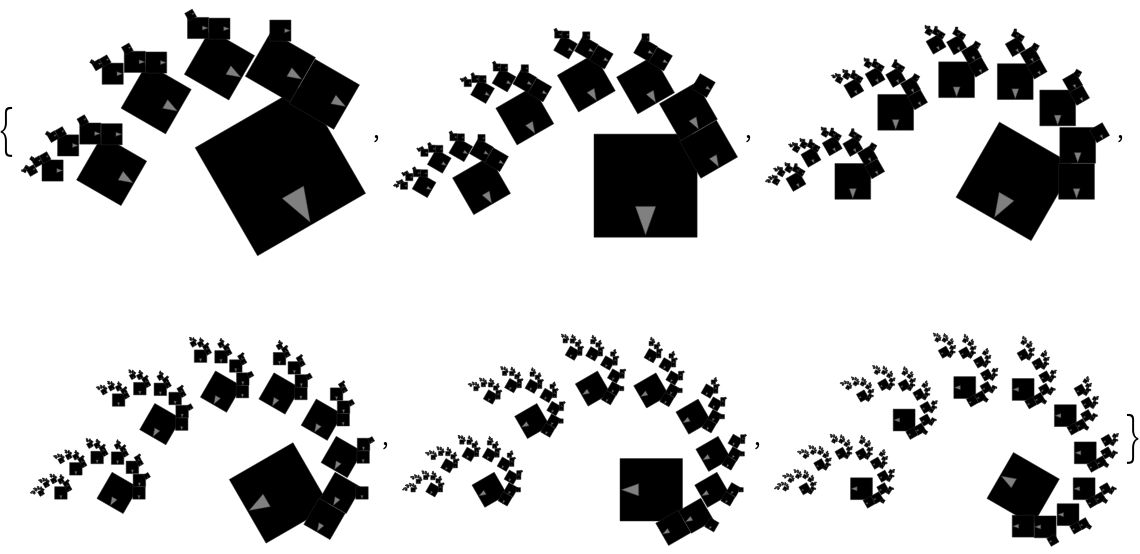Show the fifth, seventh and ninth iterations:

 In:=Out=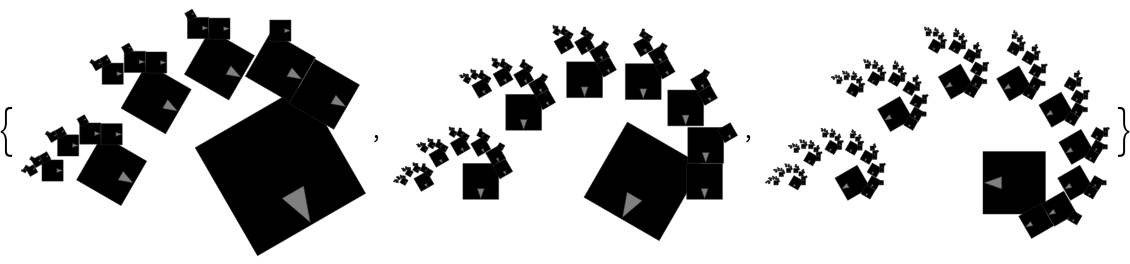Show only the fifth iteration:

 In:=Out=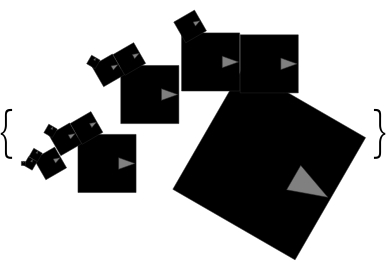Use Graphics options:

 In:=Out=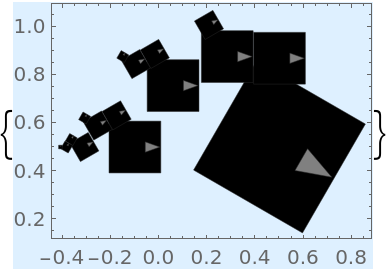### Options (1)

#### ShowMarker (1)

Set "ShowMarker"False to omit the markers in the results:

 In:=Out=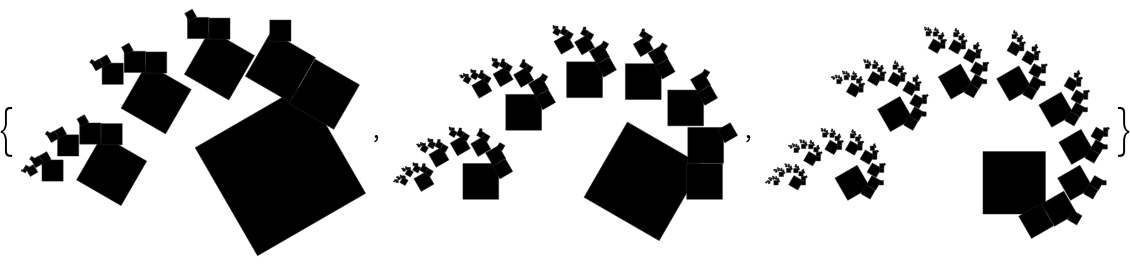### Applications (2)

The Sierpinski triangle:

 In:=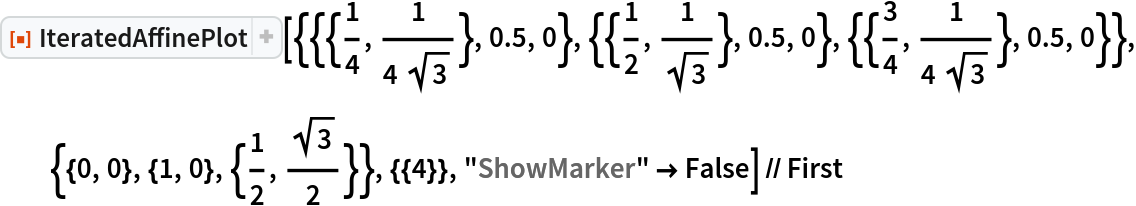Out=Compare with the result of SierpinskiMesh:

 In:=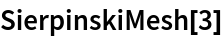Out=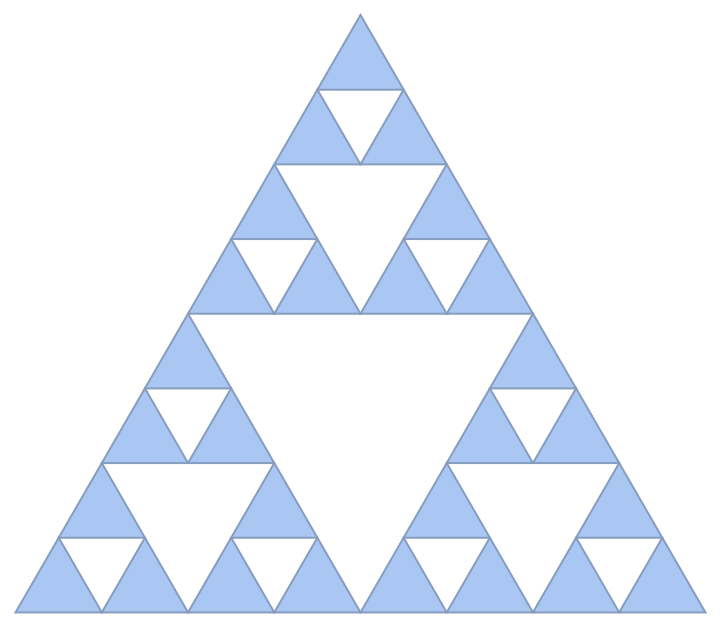The Sierpinski carpet:

 In:=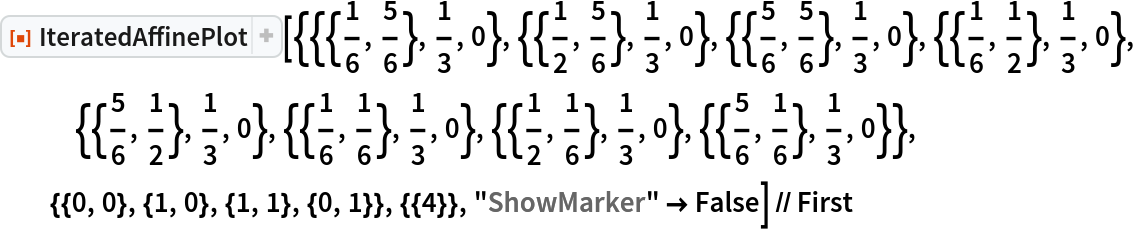Out=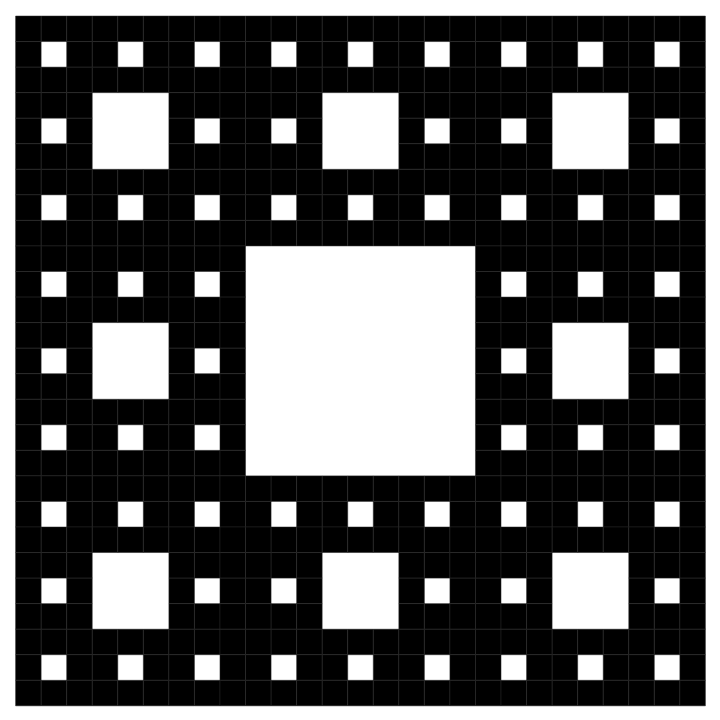Compare with the result of MengerMesh:

 In:=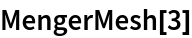Out=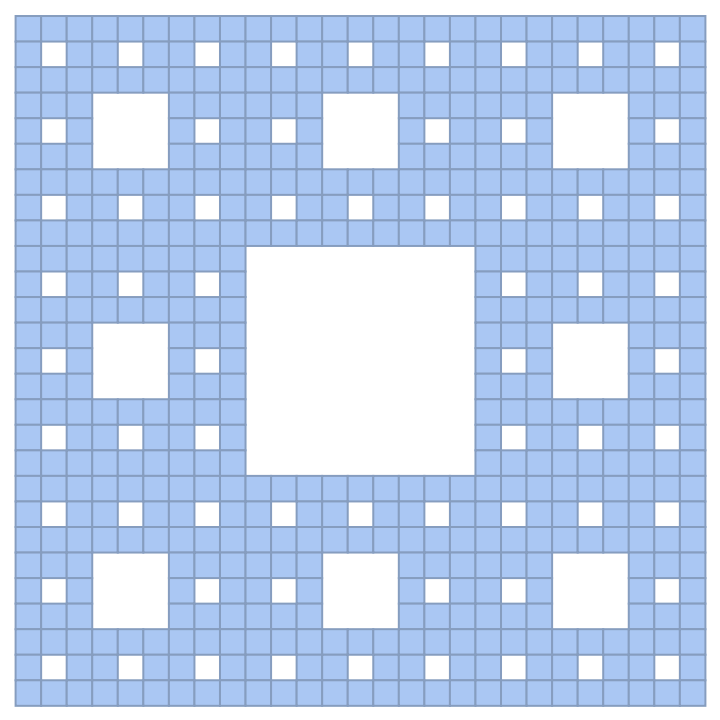## Publisher

WolframSpecialProjects

## Version History

• 1.0.0 – 17 May 2021